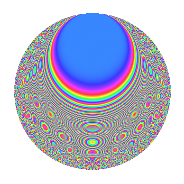# Properties

 Label 6044.2.aLevel 6044 Weight 2 Character orbit a Rep. character $$\chi_{6044}(1,\cdot)$$ Character field $$\Q$$ Dimension 126 Newform subspaces 2 Sturm bound 1512 Trace bound 3

# Related objects

## Defining parameters

 Level: $$N$$ $$=$$ $$6044 = 2^{2} \cdot 1511$$ Weight: $$k$$ $$=$$ $$2$$ Character orbit: $$[\chi]$$ $$=$$ 6044.a (trivial) Character field: $$\Q$$ Newform subspaces: $$2$$ Sturm bound: $$1512$$ Trace bound: $$3$$

## Dimensions

The following table gives the dimensions of various subspaces of $$M_{2}(\Gamma_0(6044))$$.

Total New Old
Modular forms 759 126 633
Cusp forms 754 126 628
Eisenstein series 5 0 5

The following table gives the dimensions of the cuspidal new subspaces with specified eigenvalues for the Atkin-Lehner operators and the Fricke involution.

$$2$$$$1511$$FrickeDim.
$$-$$$$+$$$$-$$$$63$$
$$-$$$$-$$$$+$$$$63$$
Plus space$$+$$$$63$$
Minus space$$-$$$$63$$

## Trace form

 $$126q - 2q^{3} - 2q^{5} + 124q^{9} + O(q^{10})$$ $$126q - 2q^{3} - 2q^{5} + 124q^{9} - 2q^{13} - 4q^{15} - 10q^{17} - 2q^{19} - 12q^{21} - 8q^{23} + 120q^{25} - 20q^{27} - 6q^{29} - 12q^{31} - 2q^{33} - 16q^{35} - 8q^{37} - 6q^{39} - 6q^{41} - 2q^{43} + 18q^{45} + 2q^{47} + 126q^{49} - 18q^{51} - 6q^{53} - 18q^{55} - 8q^{59} - 16q^{61} - 16q^{63} - 26q^{65} - 6q^{67} + 2q^{69} - 30q^{71} - 2q^{73} - 6q^{75} - 14q^{77} - 8q^{79} + 102q^{81} + 8q^{83} - 24q^{85} - 6q^{87} - 4q^{89} - 8q^{91} - 22q^{93} + 2q^{95} - 20q^{97} - 20q^{99} + O(q^{100})$$

## Decomposition of $$S_{2}^{\mathrm{new}}(\Gamma_0(6044))$$ into newform subspaces

Label Dim. $$A$$ Field CM Traces A-L signs $q$-expansion
$$a_2$$ $$a_3$$ $$a_5$$ $$a_7$$ 2 1511
6044.2.a.a $$63$$ $$48.262$$ None $$0$$ $$-7$$ $$-7$$ $$-22$$ $$-$$ $$-$$
6044.2.a.b $$63$$ $$48.262$$ None $$0$$ $$5$$ $$5$$ $$22$$ $$-$$ $$+$$

## Decomposition of $$S_{2}^{\mathrm{old}}(\Gamma_0(6044))$$ into lower level spaces

$$S_{2}^{\mathrm{old}}(\Gamma_0(6044)) \cong$$ $$S_{2}^{\mathrm{new}}(\Gamma_0(1511))$$$$^{\oplus 3}$$$$\oplus$$$$S_{2}^{\mathrm{new}}(\Gamma_0(3022))$$$$^{\oplus 2}$$

## Hecke characteristic polynomials

There are no characteristic polynomials of Hecke operators in the database